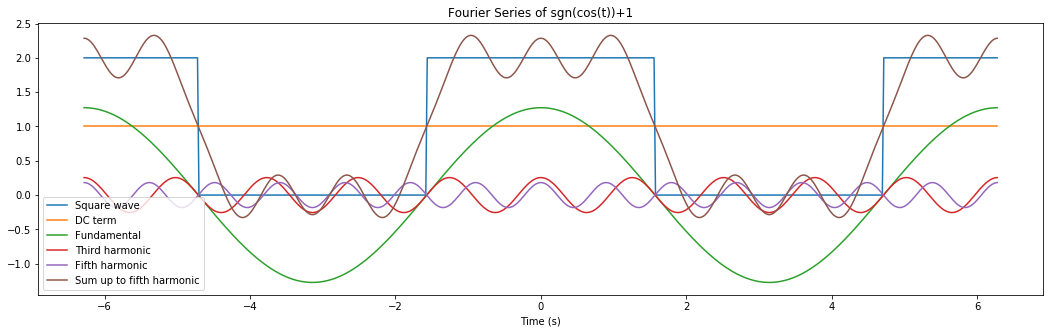# Some Complex Fourier Series Examples¶

The Complex Fourier Series for $x(t) = x(t + T_0)$ is: $$x(t) = x(t+T_0) = \sum_{n=-\infty}^{\infty} c_n e^{j2\pi nt/T_0}$$ You find the coefficients, $c_n$ by taking the inner product of both sides with $e^{j2\pi mt/T_0}$ on both sides of the equation and solving for the $c_m$ using the ortogonality of the complex exponentials. If you want more information there is a handout on fweb and there is a better copy at issuu with the whole book, and it could be useful for this class. See the chapters on Fourier Series and Integrals.

## Finding the $c_n$ for an Even Function, Like $x(t) = sgn(cos(t))+1$¶

The period, $T_0 = 2\pi$. The formula for the coefficients found using the ideas above is: $$c_m = 1/T_0 \int_{0}^{T_0} x(t)e^{-j2\pi mt/T_0} dt = 1/T_0 \int_{-T_0/2}^{T_0/2} x(t)e^{-j2\pi mt/T_0} dt$$ $$= 1/(2\pi) \int_{-\pi/2}^{\pi/2} 2e^{-j2\pi mt/(2\pi)} dt= 1/(2\pi) \int_{-\pi/2}^{\pi/2} 2e^{-j mt} dt$$ For $m=0$, $c_0 = 1$, and for $m\ne 0$, $$c_m = 2/(j2\pi m) \left[e^{jm\pi/2} -e^{-jm\pi/2} \right] = 2/(\pi m) sin(m \pi /2)$$ so the Complex Fourier Series is: $$\sum_{n=-\infty}^{\infty} c_n e^{j nt} = \sum_{n=1, 3, 5,...}^{\infty} \frac{4}{\pi n} cos(nt)$$

In :
# Python script to calculate and plot the Fourier Series for sgn(cos(t))+1
import numpy as np
import matplotlib.pyplot as plt

plt.rcParams['figure.figsize'] = [18, 5]

t = np.arange(-2*np.pi,2*np.pi, 0.02)
plt.plot(t, np.sign(np.cos(t))+1, t, np.ones(np.size(t)), t, 4/np.pi*np.cos(t), t, 4/5/np.pi*np.cos(5*t),
t, 4/7/np.pi*np.cos(7*t), t,
1 + 4/np.pi*np.cos(t) - 4/3/np.pi*np.cos(3*t) + 4/5/np.pi*np.cos(5*t) + 4/7/np.pi*np.cos(7*t))
plt.legend(['Square wave', 'DC term', 'Fundamental', 'Third harmonic','Fifth harmonic',
'Sum up to fifth harmonic'])
plt.xlabel('Time (s)')
plt.title('Fourier Series of sgn(cos(t))+1')
plt.show()## Approximating the Complex Fourier Series Coefficients using the DFT¶

A rectangular integral approximation of the integral for $c_n$ yields a useful approximation for the complex Fourier series coefficients. This is even more valuable as an interpretation of the DFT. Start by sampling (measuring) one period of $x(t)$ every $T$ seconds, with $N$ samples, so you have $x(kT)$ so $T_0 = NT$. Then use those samples to approximate the $c_n$ as follows. $$c_n = 1/T_0 \int_{0}^{T_0} x(t) e^{j2\pi nt/T_0} dt \approx 1/T_0\sum_{k=0}^{N-1} x(kT)e^{-j2\pi nkT/T_0} T$$ $$= 1/NT \sum_{k=0}^{N-1} x(kT)e^{-j2\pi nkT/NT} T$$ $$=1/N \sum_{k=0}^{N-1} x(kT)e^{-j2\pi nk/N} = 1/N X(n)$$

In [ ]: